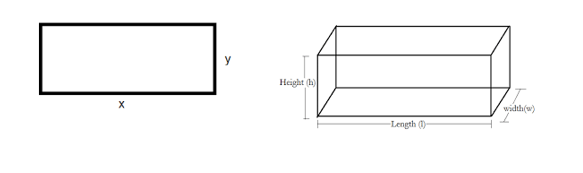# Measurement

Welcome to class!

In today’s class, we will be talking about measurement. Enjoy the class!

### Measurement#### Introduction

In life we are constantly measuring things, talking about things in terms of numbers. We talk about how many siblings we have, how many bottles of drink we took in a party, how many cars our father owns, mother measures food before they are cooked, bakers measure ingredients before using them; hence almost every one measures in one way or the other. In today’s topic, we will be learning measurement of some quantities.

#### Meaning of measurement

Measurement, the process of associating numbers with physical quantities and phenomena. Measurement means to find a number that shows the amount of something, in measurement, the size or the amount of a thing is determined. When numbers or readings are taken from different quantities. They are shown in different ways, which are called units of measurement. Examples, seconds, kilometres, centimetres etc

There are different measuring tools depending on what is to be measured.

Activity

Get two or three different sizes of orange or apple, get a twine, and determine the length of twine that would go round each of the apples or oranges.

Length and breadth are the measurements of the distance of a thing. The length of a table is the longer side while the breadth is the shorter side. The breadth of an object is also called its width. Length and breadth are measured with a ruler, meter rule, tape rule etc. the unit for measuring length and breadth is millimetre (mm), centimetre (cm) and meter (m).

In the shape below the length is represented by ‘X’ while the breadth is represented by ‘Y’.Activity

##### Area of an object

Area of an object is the total surface of that object. It is the amount of space occupied by a flat shape. Area is measured in square millimeter(mm2), square centimetre(cm2) and square meter(m2). The area of an object can be measured by multiplying together its length and breadth.Activity

In the table below find the area of each using the length and breadth provided

 Length Breadth/width Area 1 6cm 3cm 2 8cm 5cm 3 12m 2m
###### Evaluation
1. Area is the total ————— of an object
2. Area can be measured by multiplying the ———- and ——— of a shape.
3. Another name for breadth is ————-

Measurement of liquids and solids.

In our next class, we will be talking about Measurement of Liquids.  We hope you enjoyed the class.

Should you have any further question, feel free to ask in the comment section below and trust us to respond as soon as possible.

How Can We Make ClassNotesNG Better - CLICK to Tell Us💃